• 3. 血液容积 v, t=0 注射剂量 d, 血药浓度立即为 d/v. 2. 药物排除速率与血药浓度成正比比例系数 k>0) 模型假设 1. 机体看作一个房室室内血药浓度均匀 一室模型 模型建立 ? d/ c(0) 3 ? 得 由假设 -kc dt dc 2 ?...
• MATLAB 最小二乘法 线性拟合算法 线性相关系数
• MATLAB实现最小二乘法一元线性拟合，并求出预测直线的斜率与截距。
• PAGE / NUMPAGES 有一组关于MH的实验数据附件1.data已知其符合 分布请利用最小二乘法编写程序求三个参数abc的值要求利用MATLAB编程20分 解拟合两组数据MH已知 只需确定多项式系数abc根据最小二乘原则使 所求问题成为...
• 最小二乘法拟合直线程序：function linear_fit %最小二乘法拟合直线clear;clc;prompt={'Name of data file'};title='Linear_fit';lineNo=2;def={'Linearfit.dat'};outval=inputdlg(prompt,title,lineNo,def);if ...

最小二乘法拟合直线

程序：

function linear_fit %最小二乘法拟合直线clear;

clc;

prompt={'Name of data file'};

title='Linear_fit';

lineNo=2;

def={'Linearfit.dat'};

outval=inputdlg(prompt,title,lineNo,def);

if isempty(outval)==1,return,end

filename=outval{1};

x=data(:,1);

y=data(:,2);

[a,b]=linearfit(x,y);

yy=a+b*x;

func=['y=',num2str(a),'+',num2str(b),'*x']; plot(x,y,'bx','markersize',10);

hold on

plot(x,yy,'r-','linewidth',1.5)

xlabel('T(^oC)');

ylabel('R(\Omega)');

text(x(2),yy(length(yy)-1),func)

function [a,b]=linearfit(x,y)

xy=x.*y;

x2=x.^2;

x_mean=mean(x);

y_mean=mean(y);

xy_mean=mean(xy);

x2_mean=mean(x2);

b=(xy_mean-x_mean*y_mean)/(x2_mean-x_mean^2); a=y_mean-b*x_mean;

return

展开全文• MATLAB与最小二乘法拟合数据

千次阅读 2021-04-04 19:57:41
2、MATLAB基于最小二乘法原理的函数拟合 （1）常见的数据拟合直线拟合、多项式拟合、插值拟合等首先利用MATLAB中的函数来直观体验下一次拟合。 参考：http://www.qinms.com/work/nihe.html（拟合方法） h...

1、前言：学习并记录的原因

最小二乘法是一种数学优化技术，它通过最小化误差的平方和寻找数据的最佳函数匹配。

最小平方差法在误差估计、不确定度、系统辨识及预测、预报等数据处理诸多学科领域得到广泛应用的数学工具——【From百度百科】。

2、MATLAB基于最小二乘法原理的函数拟合

（1）常见的数据拟合有直线拟合、多项式拟合、插值拟合等。首先利用MATLAB中的函数来直观体验下一次拟合。

参考：http://www.qinms.com/work/nihe.html（拟合方法）

%拟合下面的数据
clear
clc
x=[9,13,15,17,18.6,20,23,29,31.7,35];
y=[-8,-6.45,-5.1,-4,-3,-1.95,-1.5,-0.4,0.2,-0.75];
%% **********************************************************%%
%{
会用polyfit函数和polyval函数
p=polyfit(x,y,n)：最小二乘法计算拟合多项式系数。
x，y为拟合数据向量，要求维度相同，n为拟合多项式次数。
返回p向量保存多项式系数，由最高次向最低次排列。
y=polyval(p,x)：计算多项式的函数值。
返回在x处多项式的值，p为多项式系数，元素按多项式降幂排序。
%}
%% 一次函数拟合
coefficient1=polyfit(x,y,1);  %用一次函数拟合曲线
y1=polyval(coefficient1,x);
%% 三次多项式函数拟合
coefficient2=polyfit(x,y,3);  %用三次函数拟合曲线
y2=polyval(coefficient2,x);
%% 三次多项式函数拟合
coefficient3=polyfit(x,y,15);  %用15次函数拟合曲线
y3=polyval(coefficient3,x);
%% 结果显示
figure('name','LSQ拟合','numbertitle','off')
subplot(2,2,1)
plot(x,y,'o')
grid on
title('原始离散数据')
h=legend('data');      %线型标识名
set(h,'Orientation','horizontal','Color', 'w','Box', 'on',...
'Location','southeast','fontsize',10,'fontweight','n','fontname',...
'times new romans','linewidth',0.5)

subplot(2,2,2)
plot(x,y,'o',x,y1,'-')
grid on
title('一次线性拟合')
h1=legend('data','poly fit');      %线型标识名
set(h1,'Orientation','vertical','Color', 'w','Box', 'on',...
'Location','southeast','fontsize',10,'fontweight','n','fontname',...
'times new romans','linewidth',0.5)

subplot(2,2,3)
plot(x,y,'o',x,y2,'-')
grid on
title('三次多项式拟合')
h2=legend('data','poly fit');
set(h2,'Orientation','vertical','Color', 'w','Box', 'on',...
'Location','southeast','fontsize',10,'fontweight','n','fontname',...
'times new romans','linewidth',0.5)

subplot(2,2,4)
plot(x,y,'o',x,y3,'-')
grid on
title('十五次多项式拟合')
h3=legend('data','poly fit');
set(h3,'Orientation','vertical','Color', 'w','Box', 'on',...
'Location','southeast','fontsize',10,'fontweight','n','fontname',...
'times new romans','linewidth',0.5)
%% 插值拟合***************************************************************%%
figure('name','插值拟合','numbertitle','off')
x2 = 6:0.01:35;
%{
MATLAB中的插值函数为interp1，其调用格式为：  yi= interp1(x,y,xi,'method')
其中x，y为插值点，yi为在被插值点xi处的插值结果；x,y为向量，
'method'表示采用的插值方法，MATLAB提供的插值方法有几种：
'nearest'是最邻近插值， 'linear'线性插值； 'spline'三次样条插值；
'pchip'立方插值．缺省时表示线性插值
注意：所有的插值方法都要求x是单调的，并且xi不能够超过x的范围。
%}
subplot(2,2,1);
plot(x,y,'ko');
title('原始离散数据')
grid on

y4 = interp1(x,y,x2,'spline');
subplot(2,2,2);
plot(x,y,'ko',x2,y4,'r')
title('spline插值法拟合')
grid on

y5 = interp1(x,y,x2,'linear');
subplot(2,2,3);
plot(x,y,'ko',x2,y5,'r')
title('linear插值法拟合')
grid on

y6 = interp1(x,y,x2,'pchip');
subplot(2,2,4);
plot(x,y,'ko',x2,y6,'r')
title('pchip插值法拟合')
grid on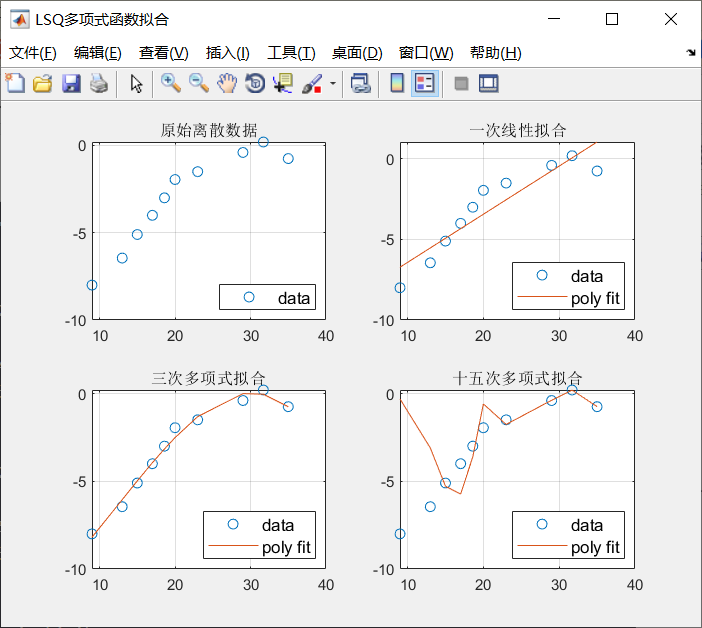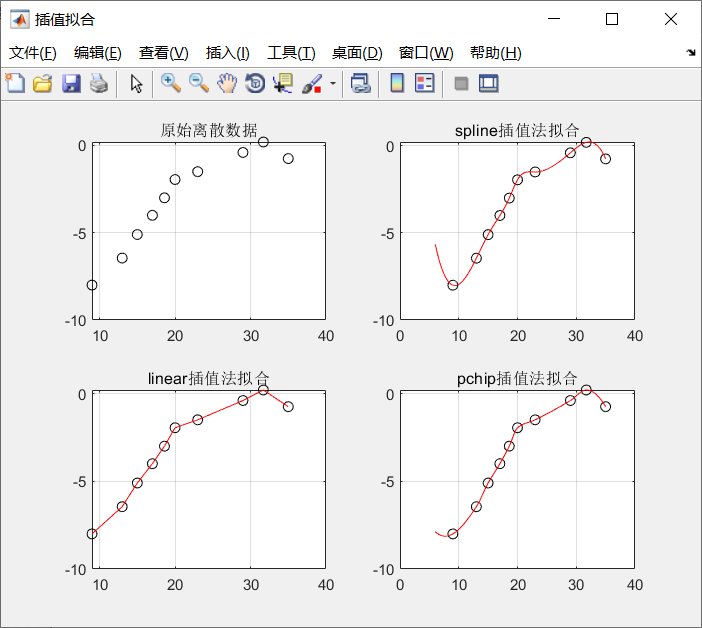3、对非线性函数进行拟合（辨识参数？）

假设我们大概知道某数学模型的函数为：y=a*log（x）+b；在大致模型参数进行数据采样【如代码中的假设使用的参数a和b分别为-1.5、-10】，如何确定较准确的参数a和b。

%matlab的最小二乘拟合函数对非线性函数进行拟合
% [q r] = lsqcurvefit(fun, q_0, xdata, ydata);
% 输入参数：
% fun：需要拟合的函数，假定有n个需要拟合的参数，那么 q = [q1,q2,...,qn]
% q_0：表示用户给定的一个起始点
% xdata：函数的自变量
% ydata：函数的因变量
% 输出参数：
% q：表示求解得到的最优参数
% r：表示最小二乘的目标函数值，即残差。
x = 1:1:100;
a = -1.5;
b = -10;
y = a*log(x)+b;
yrand = y + 0.5*rand(1,size(y,2));% 在模拟模型参数的偏差下的采样数据
plot(x,yrand,'ro');
%% 最小二乘拟合
xf=log(x);
yf=yrand;
f=inline('b(1)+b(2).*x','b','x');% 内联函数: f(b,x) = b(1)+b(2).*x
[q,r]=lsqcurvefit(f,[1,0],xf,yf);%起点[1,0]拟合
plot(x,yrand,'ro','LineWidth',1) %绘制采样点
hold on;
%% 绘制拟合曲线
yn = q(1)+q(2)*log(x);
hold on;
grid on
plot(x,y,'c','LineWidth',2);%原函数
plot(x,yn,'b','LineWidth',2);%拟合线
%% 设置Legend
hleg = legend(['按原函数(y=' num2str(b,3) '+' num2str(a,3) 'ln(x)' ')采样数据'],...
['原函数为(y=' num2str(b,3) '+' num2str(a,3) 'ln(x)' ')的曲线'],...
['拟合结果：y=' num2str(q(1),3) '+' num2str(q(2),3) 'ln(x)'],...
'Location','NorthEast');%本身不能设置字体的大小,需要通过set进行设置
set(hleg,'FontSize', 10, 'FontAngle','italic','FontWeight','bold',...
'TextColor',[.6,.2,.1],'Color',[1,1,1]);%Color为设置坐标的背景颜色
title('最小二乘拟合：y=a+b*ln(x)','Color','k','FontSize',12);
xlabel('x','Color','k','FontSize',10);%横轴标题
ylabel('y','Color','k','FontSize',10);%纵轴标题

%% 保存图像
set(1, 'InvertHardCopy', 'off');%设置的背景色有效,如果为on则图形不保存背景色，maltab 默认为 on
filename = 'lnx';
print(1, '-djpeg', filename);%其他格式 -djpeg,-dpng,-dbmp,-dtiff,-dgif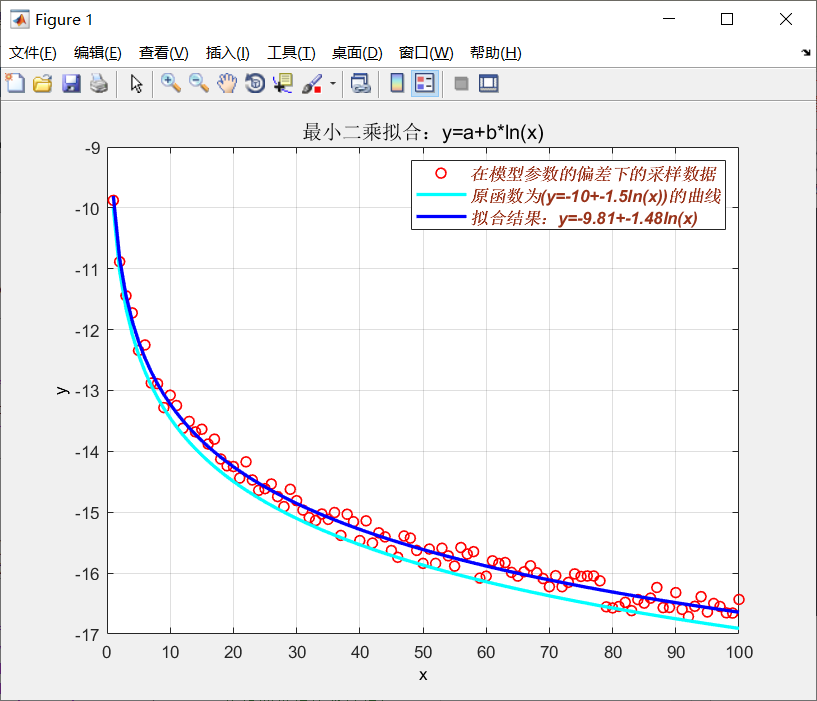4、尝试编写的直线拟合：

https://zhidao.baidu.com/question/623179322094537004.html （很好的方便理解的一个回答，如下过程）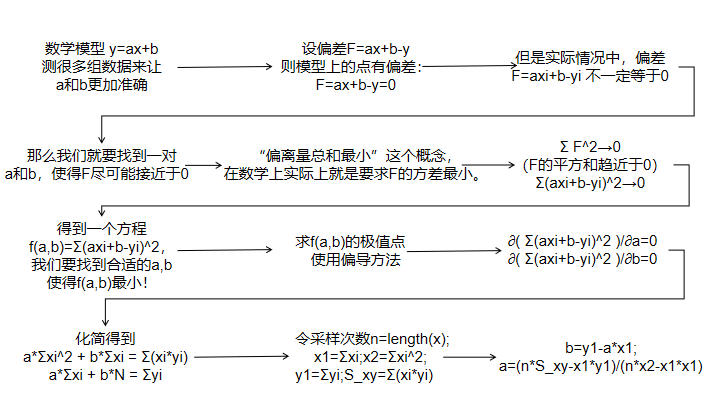%% 拟合的数据
clear
clc
x=[9,13,15,17,18.6,20,23,29,31.7,35];
y=[-8,-6.45,-5.1,-4,-3,-1.95,-1.5,-0.4,0.2,-0.75];
%%  参考自编的一次直线拟合
n=length(x);        % 变量个数
x2=sum(x.^2);       % 求Σ(xi^2)
x1=sum(x);          % 求Σ(xi)
S_xy=sum(x.*y);     % 求Σ(xi*yi)
y1=sum(y);          % 求Σ(yi)
a=(n*S_xy-x1*y1)/(n*x2-x1*x1);      %解出直线斜率
b=(y1-a*x1)/n;                      %解出直线截距b=(y1-a*x1)/n
%作图
% 先把原始数据点用蓝色十字描出来
figure('name','直线拟合','numbertitle','off')
% 用红色绘制拟合出的直线
px=linspace(0 ,35,12);%0到35等线性间距的分为12份
py=a*px+b;
plot(x,y,'o',px,py,'r');
grid on
h1=legend('data','poly fit');      %线型标识名
set(h1,'Orientation','vertical','Color', 'w','Box', 'on',...
'Location','southeast','fontsize',10,'fontweight','n','fontname',...
'times new romans','linewidth',0.5)
a,b
%% 对比polyfit函数的直线拟合参数
coefficient1=polyfit(x,y,1)

一次直线拟合和polyfit的一次直线拟合得到的参数是相同的。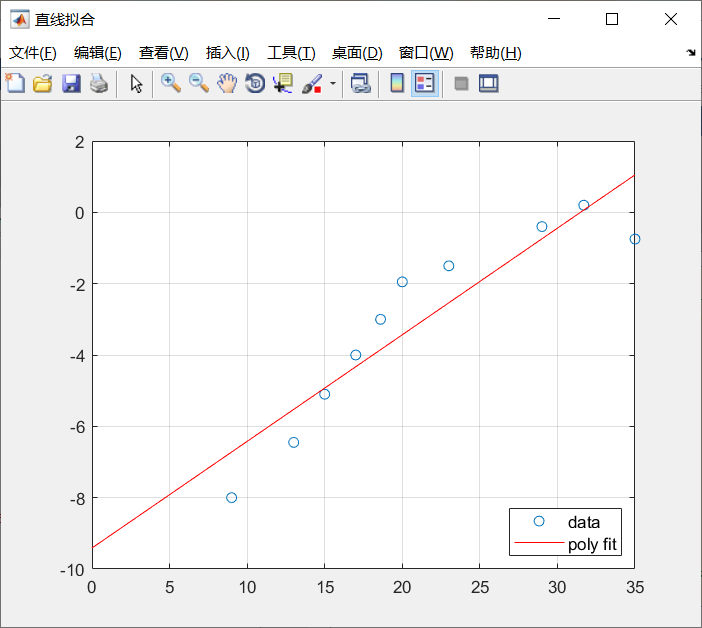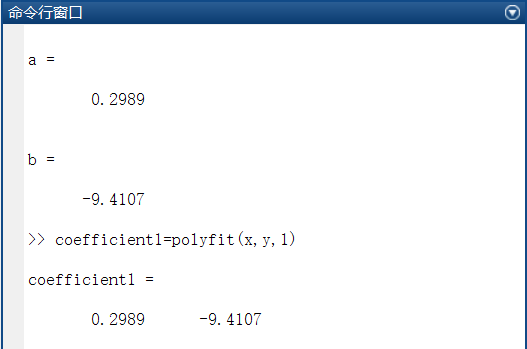5、MATLAB工具箱cftool

参考：https://blog.csdn.net/qq_36607894/article/details/99996277（有些拟合结果评价的内容）

%% 工具箱在命令行直接运行
sftool%老版本
cftool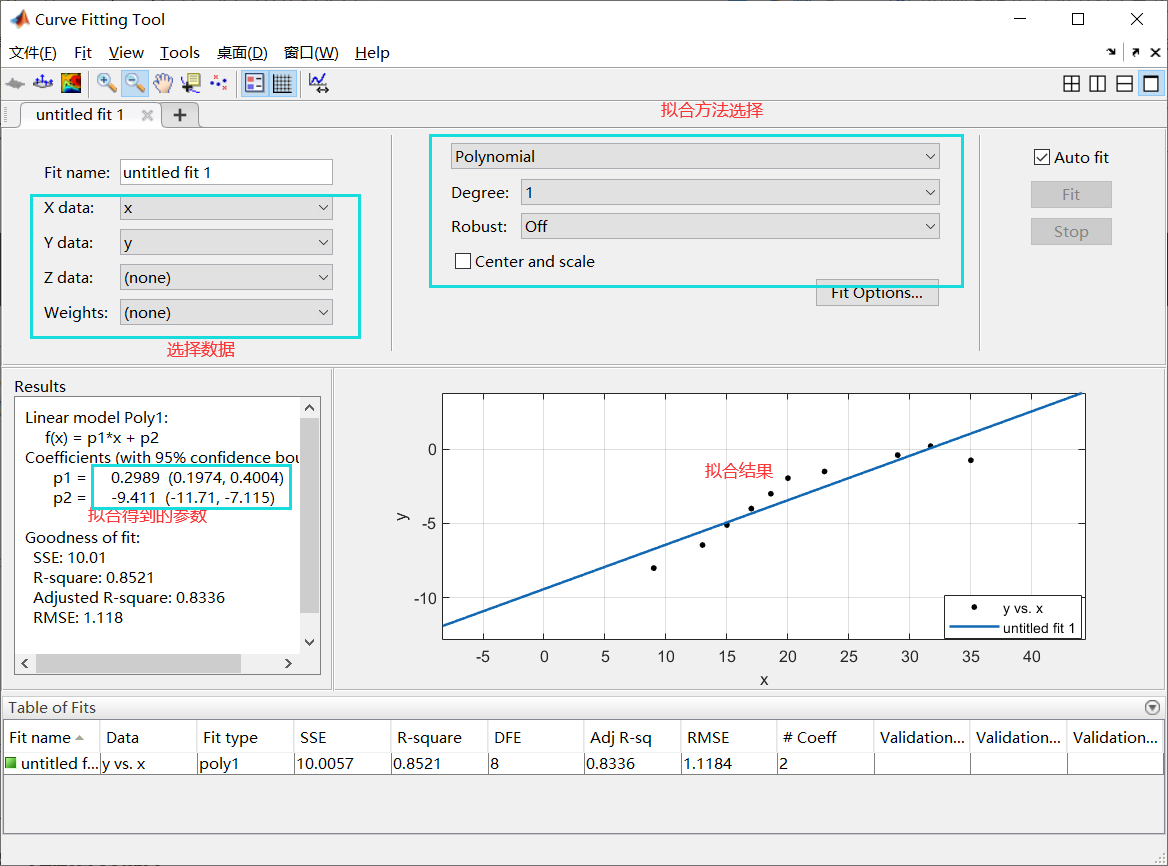后记：先记录到这里，后面有的再更。

其他参考：（1）Python版本：https://www.jianshu.com/p/af0a4f71c05a

（2）最小二乘法的本质知乎回答：https://www.zhihu.com/question/37031188

（4）matlab 最小二乘拟合平面的方法：https://blog.csdn.net/qq_36686437/article/details/109552543

展开全文matlab 最小均方误差
• %得到子块里白色像素点拟合得到的直线的斜率k和截距b（调用zikuai函数） X{count}=x; %保存子块里所有白色像素的x坐标 Y{count}=y; %保存子块里所有白色像素的y坐标 K(count)=k; %保存子块里拟合得到的直线的斜率k ...

一、源代码

function chengxu()
%1步
close all
I=rgb2gray(I);    %彩色图像转换成灰度图

% I=im2bw(I);      %二值化
I=edge(double(I)); %检测图像的边缘
figure
imshow(I)          %显示边缘检测的结果

%2[m,n]=size(I);     %计算图像的尺寸

M=3;             %定义X方向分割的块数
N=3;             %定义Y方向分割的块数
mm=floor(m/M);   %子块行的长度
nn=floor(n/N);   %子块列的长度
count=1;         %计数器
figure
for i=1:M
for j=1:N
A=I((i-1)*mm+1:i*mm,(j-1)*nn+1:j*nn);    %分割原图像，得到一个子块
subplot(M,N,count)
imshow(A)               %显示一个子块
zuoshangjiao=[(i-1)*mm+1 (j-1)*nn+1];  %子块左上角的坐标
[x,y,k,b]=zikuai(A,zuoshangjiao);      %得到子块里白色像素点拟合得到的直线的斜率k和截距b（调用zikuai函数）
X{count}=x;       %保存子块里所有白色像素的x坐标
Y{count}=y;       %保存子块里所有白色像素的y坐标
K(count)=k;       %保存子块里拟合得到的直线的斜率k
B(count)=b;       %保存子块里拟合得到的直线的截距b
count=count+1;    %计数器加1，进行下一个子块的计算
end
end

二、运行结果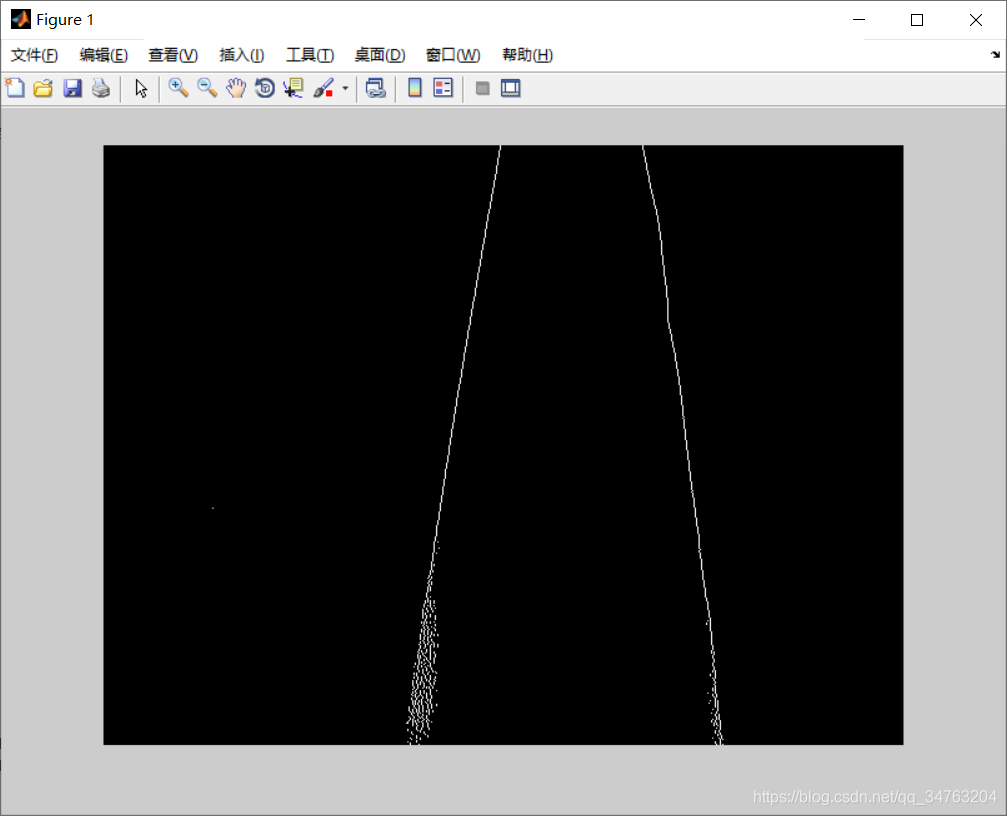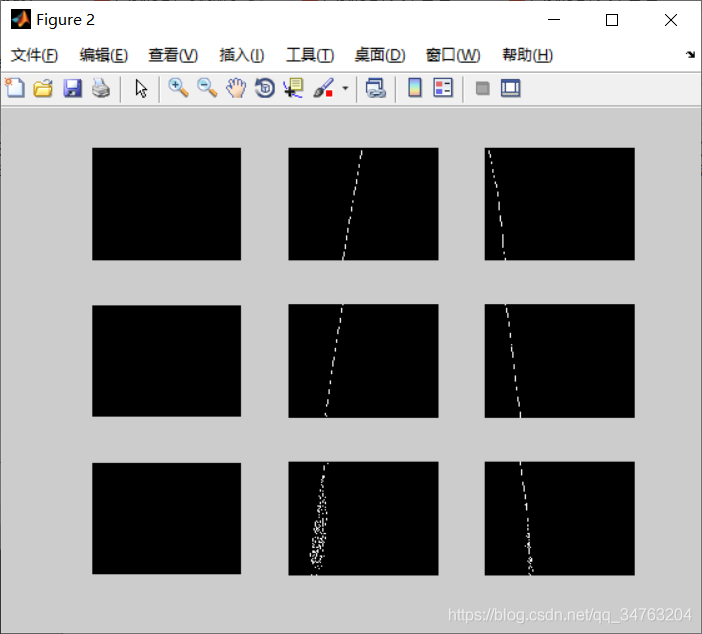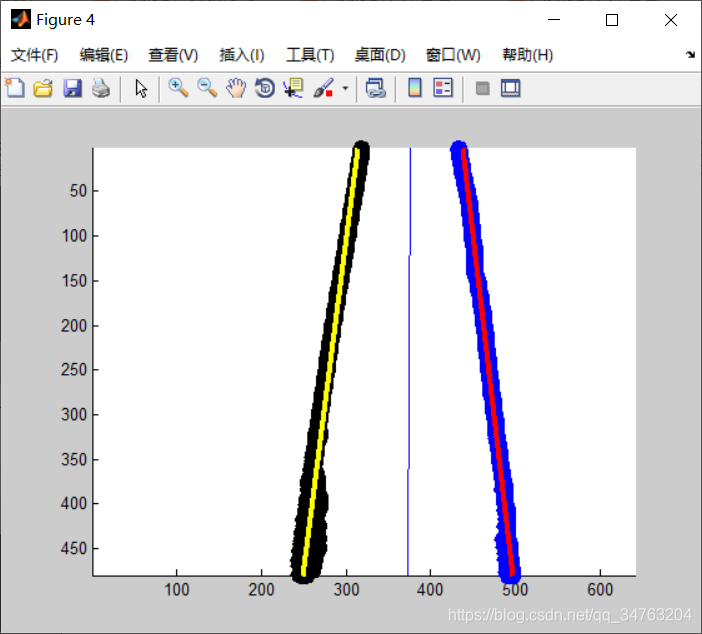三、备注

版本：2014a

展开全文• 针对在直线拟合中,因变量选取不同拟合的结果有差异现象,提出采用整体最小二乘法进行直线拟合。文章在分析直线方程特点的基础上,采用EIV模型描述直线方程,在解算中根据系数矩阵的特点应用QR分解分为将方程两部分,采用...
• 最小二乘法曲线拟合以及Matlab实现 在实际工程中，我们常会遇到这种问题：已知一组点的横纵坐标，需要绘制出一条尽可能逼近这些点的曲线（或直线），以进行进一步进行加工或者分析两个变量之间的相互关系。而获取这...最小二乘法
• 提供测试用例，输入三维离散点空间坐标，可以直接获得最小二乘法的空间拟合直线，并可以求出每个离散点到空间直线的距离，方便剔除偏离较大的离散点
• 最小二乘法所用的函数： p = polyfit(x,y,1);x,y为函数参数，1为一阶函数， 输出拟合直线的方程： p1=poly2sym(p) 初步代码如下： 简单应变片传感器数据集： x = [20,40,60,80,100,120,140,160,180,200]; y = ...

最小二乘法所用的函数：

p = polyfit(x,y,1);x,y为函数参数，1为一阶函数，

输出拟合直线的方程：

p1=poly2sym(p)

初步代码如下：

简单应变片传感器数据集：

x = [20,40,60,80,100,120,140,160,180,200];
y = [9.9,20,30.3,40.2,50.3,60.4,70.4,80.3,90.6,100.7];
p = polyfit(x,y,1);
x1 = 0:0.01:200;
y1 = polyval(p,x1);
subplot(1,2,2);
plot(x,y,'k^:',x1,y1,'k')
title('黑线为最小二乘法拟合')
%  grid on
p1=poly2sym(p)

展开全文matlab
• 利用曲线拟合的线性最小二乘法求被逼近函数f(x)在点x=55处的近似值，并画出实验数据和直线。 代码实现 X=[0,10,20,30,40,50,60,70,80,90]; Y=[68,67.1,66.4,65.6,64.6,61.8,61.0,60.8,60.4,60]; n=length(X);%matlab
• MATLAB实现最小二乘法

万次阅读 多人点赞 2019-03-31 20:40:32
文章仅为创作者的观点，可与转载者讨论 最小二乘法         最小二乘法（又称最小平方法）是一种数学优化技术。它通过最小化误差的平方和寻找数据的最佳函数匹配。     &nb...最小二乘法 matlab
• Matlab曲线拟合 最小二乘法 polyfit2009-04-07 19:04曲线拟合已知离散点上的数据集，即已知在点集上的函数值，构造一个解析函数(其图形为一曲线)使在原离散点上尽可能接近给定的值，这一过程称为曲线拟合。...
• 最小二乘法拟合直线 程序 function linear_fit % 最小二乘法拟合直线 clear; clc; prompt={'Name of data file'}; title='Linear_fit; lineNo=2; def={'Linearfit.dat'}; outval=inputdlg(prompt,title,lineNo,def; ...
• % 用最小二乘法进行直线拟合 plot(X, Y, 'o') xlabel('x') ylabel('y') hold on % a = (X.' * X).^(-1) * (X.') * Y x = mean(X); % X的均值 y = mean(Y); % Y的均值 b_0 = (X-x) * ((Y-y).') / ((X-x) * ((X-x).'))...
• 最小二乘法可以帮助我们在进行线性拟合时，如何选择“最好”的直线。要注意的是，利用实验数据进行拟合时，所用数据的多少直接影响拟合的结果，从理论上说，数据越多，效果越好，即所估计的直线方程越能更好地反映...二次函数回归方程 最小二乘法建模例题
• 用表1-1中的世界人口统计数值估计1980年的人口，求最佳最小二乘法数值估计：表 1-1：年人口19603 039 585 53019703 707 475 88719905 281 653 82020006 079 603 571(a) 直线；(b) 抛物线。它们都通过这些数据点，并...
• MATLAB之最小二乘法

千次阅读 2020-04-15 16:49:28
拟合曲线为y=ax+b,根据条件可写出线形方程组为： [x1 1;x2 1;...;x3;1]*[a;b]=[y1;...;yn]即A*[a;b]=[y2;...;yn] 因为A不是方阵，无法求逆。故做如下变形： 方法一： 方法二： 对矩阵A做QR分解 二...matlab 算法 线性代数
• 帮朋友利用实验数据画图时，发现MatLab的确是画图的好工具，用它画的图比Excel光滑、精确。利用一组数据要计算出这组数据对应的函数表达式从而得到相应图像，MatLab的程序如下：x=[1 5 10 20 30 40 60 80]y=[15.4 ...
• 最小二乘法入门（Matlab直线和曲线拟合）

万次阅读 多人点赞 2019-02-28 14:13:47matlab
• matlab最小二乘法的非线性参数拟合首先说一下匿名函数：在创建匿名函数时，Matlab记录了关于函数的信息，当使用句柄调用该函数的时候，Matlab不再进行搜索，而是立即执行该函数，极大提高了效率。所以首选匿名函数。...
• 曲线拟合最小二乘法matlab举例，介绍了如何用MATLAB对实验所得数据，运用最小二乘法画出曲线，并计算线性度、非线性误差。
• k k 1 matlab最小二乘法拟合 [a,Jm...卡尔曼MATLAB-最小二乘法例子的代码_信息与通信_工程科技_专业资料。 Ts...最小二乘法曲线拟合原理及matlab实现_数学_自然科学_专业资料。曲线拟合(c...[10x10 double] df: 22 ...
• 最小二乘法直线拟合及其Matlab实现

万次阅读 多人点赞 2014-12-10 16:47:57
最小二乘法，通常用在我们已知数学模型，但是不知道模型参数的情况下，通过实测数据，计算数学模型，例如，在题目中，数学模型就是直线方程y=ax+b，但是不知道直线方程的a和b。   本来呢，我们只需要两组（xi,yi）...最小二乘法 Matlab
• 最小二乘法的基本原理和多项式拟合matlab实现 最小二乘法的基本原理和多项式拟合一、最小二乘法的基本原理从整体上考虑近似函数 )(xp同所给数据点 ),(iyx(i=0,1,…,m)误差iiiyxpr)((i=0,1,…,m) 的大小，......

matlab拟合最小二乘法直线matlab 订阅